# GraphSketch.com - ParametricClick here to download this graph.

## Mode:

Functions Parametric

## Settings:

• X Range: to
• Y Range: to
• T Range: to
• X Tick Distance:
• Y Tick Distance:
• Label Every: X ticks
• Label Every: Y ticks
• Show Grid:
• Bold Labeled Gridlines:
• Function Width: pixels
• Image Size: by pixels

Beyond simple math and grouping (like "(x+2)(x-4)"), there are some functions you can use as well. Look below to see them all. They are mostly standard functions written as you might expect. You can also use "pi" and "e" as their respective constants.

Please note: You should not use fractional exponents. For example, don't type "x^(1/3)" to compute the cube root of x. Instead, use "root(x,3)".

When you want a quick graph of a function, you can just go to http://graphsketch.com/[function], like http://graphsketch.com/sin(x). You can even separate multiple equations with commas, like http://graphsketch.com/sin(x),x^2.

For more information on GraphSketch (how it works, etc.), see my blog post on it.

## Support GraphSketch:GraphSketch is provided by Andy Schmitz as a free service. Buying a poster from posters.lardbucket.org helps support GraphSketch and gets you a neat, high-quality, mathematically-generated poster. If you're interested, take a look. Thanks!

## Functions:

 To get: Type: To get: Type: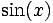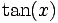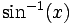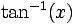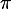sin(x) cos(x) tan(x) asin(x) acos(x) atan(x) pi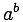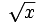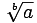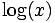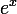a^b sqrt(x) root(a,b) log(x) ln(x) exp(x) e